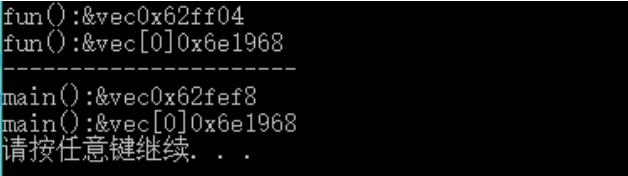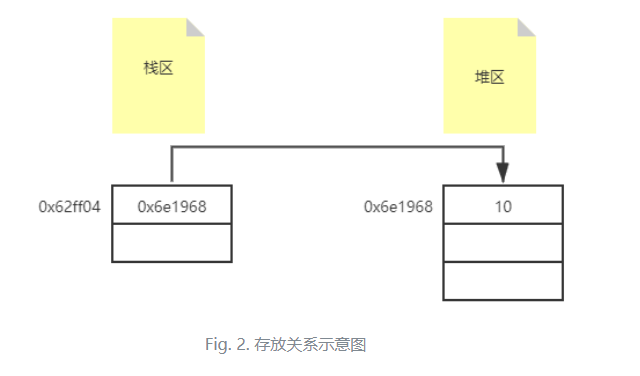# 为什么 C++ 可以返回 Vector 局部变量

0收藏

vector与动态数组性质相同。

C++语言中，所有new和malloc创建的变量均存放在堆区，这已经是一个共识。但是鲜为人知的是，STL库中的容器虽没有经过这两个关键字创建，但同样是存放在堆区。这与动态数组性质相同。如果从汇编角度观察便会发现，容器均调用了allocator来创建。这里便不展开多言。

``````#include <iostream>
#include <vector>

using namespace std;

// 返回一个vector变量，并打印vec的地址和存放在容器中第一个数据的首地址
vector<int> fun() {
vector<int> vec;    // 创建vector
vec.push_back(10);   // 添加一个元素

cout << "fun():&vec" << &vec << endl;
cout << "fun():&vec" << &vec << endl;

return vec;   // 返回vector

}

int main() {
vector<int> vec;   // 创建一个vector
vec = fun();      // 接收传来的参数

cout << "----------------------" <<endl;

cout << "main():&vec" << &vec << endl;
cout << "main():&vec" << &vec << endl;

system("pause");
return 0;
}``````Fig. 1. 运行现象C++本身提供了一个clear()函数，可以清除容器中所有元素。但是此函数并不能释放掉vector的capacity空间。如果想释放掉所有空间，需使用swap()函数，使用方法如下：

vector<int>().swap(vec);   // vec便是需要清空的容器。